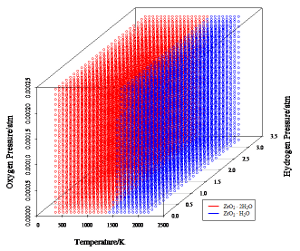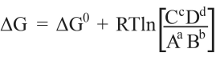# Stability of Zirconium Cladding Under PWR Conditions

## Marie Maher September 26, 2012

### Submitted as coursework for PH241, Stanford University, Winter 2012Fig. 1: Stability Window of Zirconium under PWR Conditions.

## Motivation

After an initial investigation into the thermodynamic stability of water under conditions found in a nuclear reactor, I became interested in how other materials might be modeled under the same conditions. Perhaps the most demanding material is the cladding for the fuel rods. The conventional choice is either pure zirconium or a zirconium alloy.  Modeling alloy corrosion is a non-trivial task; however, pure zirconium is a somewhat more tractable problem. In fact, under average conditions (i.e. not in a nuclear reactor), the model has been established since the 1940s.  This method is extensible to more harsh conditions, but it requires some complex calculations which will not be explicitly presented here; however, the general procedure will be outlined and interested readers are referred to Pourbaix's "Atlas of Electrochemical Equilibria in Aqueous Solutions".

## Some Important Background for Non-Scientists

The method for calculation hinges on the concept of Gibbs free energy. If a system is stable and no net reaction is occuring, the Gibbs energy for that system will be 0 (equilibrium).  Every system tends towards equilibrium, and the direction of the required change is described by the sign of the Gibbs energy, relative to some standard reference. Typically the reaction in question is written in the form aA + bB = cC + dD, where A and B are reactants, C and D are products, and the lowercase letters represent the coefficients required to balance the equation. In this convention, the change in Gibbs energy is calculated as the Gibbs energy of formation of the products minus the energy of formation of the reactants. This value is the "standard" by which we measure equilibrium, according to the equation:where G0 is the standard gibbs energy, R is the universal gas constant, T is the Kelvin temperature.

When the equilibrium concentrations of A,B,C,and D are present (i.e. the system is at equilibrium), then G is 0, as the logarithmic term exactly cancels out the standard Gibbs energy. However, in cases where the concentrations are not at their equilibrium values, the sign of G determines in which direction the reaction will proceed. if the calculated G is negative, then the reaction will proceed towards the products. If, however, G is positive, then the reaction will run from the products side to the reactants side. Through these calculations, one can calculate whether a given material is stable or will dissolve into solution.

## General Method

First, the soluble forms of the material in question must be determined (i.e. any corrosion products). These species will all be in equilibrium with each other in solution, and so their equilibrium concentrations must be determined for the set of conditions being modeled.

Second, the stabilities of all solid phases must be determined at the given set of conditions. This means that each phase must be evaluated for stability versus every other phase to determine the most stable phase. Once the most stable phase is determined, it is necessary to determine whether that phase is stable with respect to the solution equilibrium (i.e. if it will corrode/dissolve or not). Generally speaking, non-noble metals are not stable in water; however, their oxide layers are. Therefore the typical process is to determine which oxide is most stable (if any), and then determine if it will dissolve into the solution or not. In practice, however, there are frequently many oxide phases and many soluble species, and the calculations require computational assistance if they are to be done in any reasonable time frame over a large reaction space (obviously, this becomes more significant as the calculation becomes more fine-grained).

Finally, once the stability regimes have been determined, the results can be plotted with respect to the variables in the reaction space. Unfortunately, humans have a difficult time visualizing more than three dimensions, so the three most relevant parameters must be selected, in this case, temperature, hydrogen pressure, and oxygen pressure. For the diagram below, the total concentration of zirconium ions in solution is 1 ppm and the pH is 6.8. 

## The Stability of Zirconium

The graph shows two distinct stability regimes, where zirconium oxide exists in either the dihydrate or monohydrate state. This forms an apparently effective passivation layer all the way up to the melting point of zirconium. However, this analysis does have one key weak point that is worth discussing. The solubility of zirconium oxide is very low and consequently is difficult to accurately determine. Also, solubility is a function of temperature, but it is difficult to know how much it would change at such high temperatures when water doesn't behave as normal water does. Therefore, without better estimation of the solubility of the passive oxide layer, the above graph does contain some uncertainty. Nevertheless, the analysis is extensible over a range of conditions and is helpful in illustrating trends in stability.

© Marie Maher. The author grants permission to copy, distribute and display this work in unaltered form, with attribution to the author, for noncommercial purposes only. All other rights, including commercial rights, are reserved to the author.

## References

 D. Taylor, "Corrosion Resistant Cladding for Nuclear Fuel Rods." U.S. Patent 5,026,516, 25 Jun 91.

 M. Pourbaix, Atlas of Electrochemical Equilibria in Aqueous Solutions (Pergamon, 1966), p. 223.

 D. A. McQuarrie and J. D. Simon, Physical Chemistry: A Molecular Approach (University Science Books, 1997) p. 1061.

 W. Bilanin et al., "LWR Water Chemistry Guidelines," Prog. Nucl. Energy 20, 1 (1987).